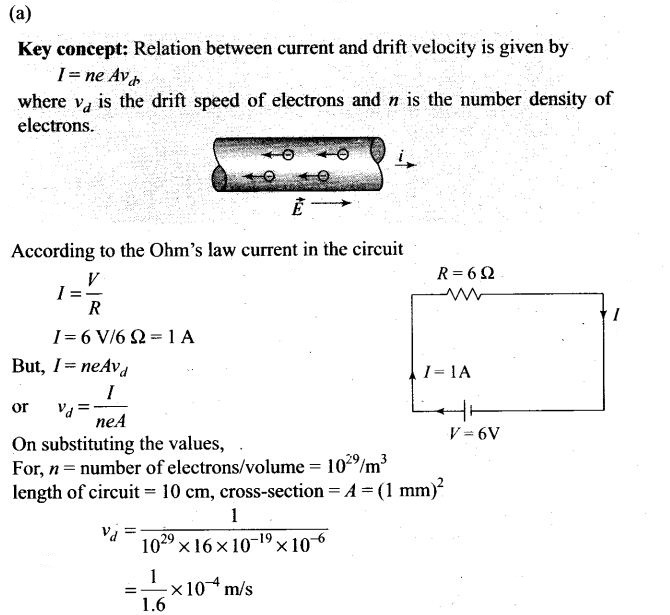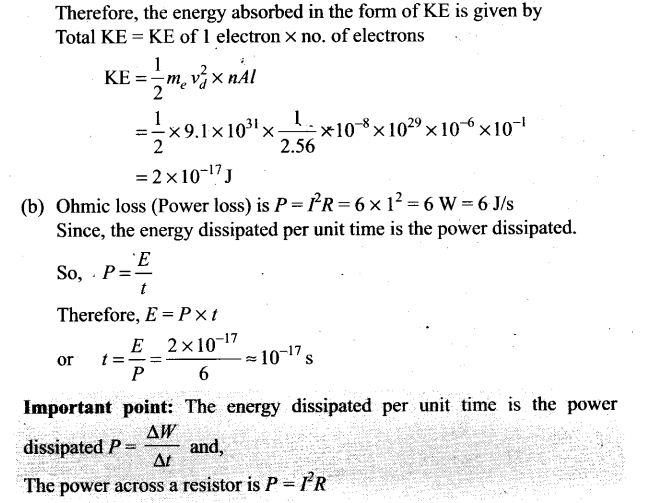PHP error in Ad Inserter block 1 - Block 1
Warning: Undefined variable \$link

# Current Electricity NCERT Exemplar Problems Solutions Physics

## NCERT Exemplar Problems Class 12 Physics Chapter 3 Current Electricity

Multiple Choice Questions (MCQs)

Single Correct Answer Type
Question 1. Consider a current carrying wire (current 7) in the shape of a circle. Note that as the current progresses along the wire, the direction of j (current density) changes in an exact manner, while the current I remain unaffected. The agent that is essentially responsible for is
(a) source of emf
(b) electric field produced by charges accumulated on the surface of wire
(c) the charges just behind a given segment of wire which push them just the right way by repulsion
(d) the charges ahead
Solution: (b)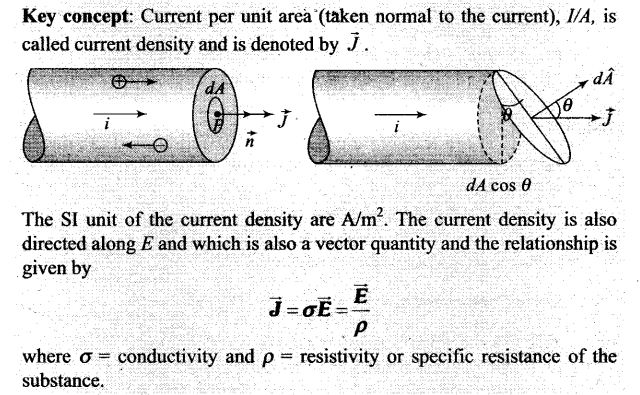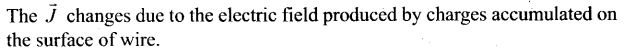Question 2.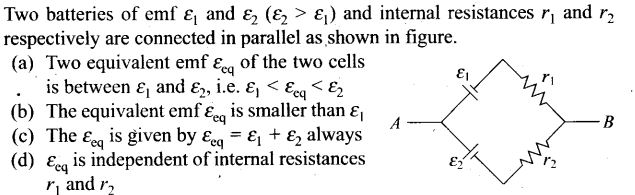Solution: (a) The equivalent emf of this combination is given by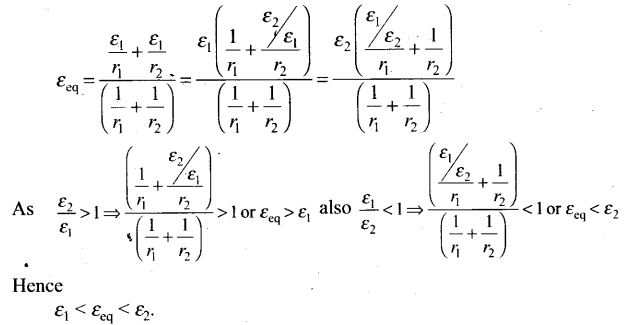Question 3. A resistance R is to be measured using a meter bridge, a student chooses the standard resistance S to be 100 Ω He finds the null point at l1 = 2.9 cm. He is told to attempt to improve the accuracy. Which of the following is a useful way?
(a) He should measure l1, more accurately
(b) He should change S to 1000 Ω and repeat the experiment
(c) He should change S to 3 Ω and repeat the experiment
(d) He should have given up hope of a more accurate measurement with a meter bridge
Solution: (c)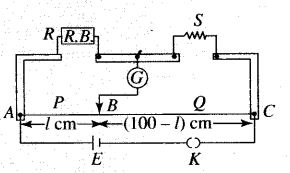Key concept: In this problem, the concept of balanced Wheatstone bridge is to be used.
Condition of balanced wheatstone bridge: The bridge is said to be balanced if the ratio of the resistances in same branch is equal R/S =l1/(100-l1)
Wheatstone bridge is an arrangement of four resistances which can be used to measure one unknown resistance of them in terms of rest.
The percentage error in R can be minimised by adjusting the balance point near the middle of the bridge, i.e., when /, is close to 50 cm. This requires a suitable choice of S.
Since , R/S =l1/(100-l1)
Since here, R : S = 2.9 : 97.1
then the value of S is nearly 33 times to that of R. In order to make this ratio 1:1, it is necessary to reduce the value of S nearly 1/33 times, i.e., nearly 3 Ω.

Question 4. Two cells of emfs approximately 5 V and 10 V are to be accurately compared using a potentiometer of length 400 cm.
(a) The battery that runs the potentiometer should have voltage of 8 V.
(b) The battery of potentiometer can have a voltage of 15 V and R adjusted so that the potential drop across the wire slightly exceeds 10 V.
(c) The first portion of 50 cm of wire itself should have a potential drop of 10 V.
(d) Potentiometer is usually used for comparing resistances and not voltages.
Solution: (b)
Key concept: The potential drop along the wires of potentiometer should be greater than emfs of cells.
In a potentiometer experiment, the emf of a cell can be measured if the potential drop along the potentiometer wire is more than the emf of the cell to be determined. Here, values of emfs of two cells are given as 5 V and 10 V, therefore, the potential drop along the potentiometer wire must be more than 10 V.

Question 5. A metal rod of length 10 cm and a rectangular cross-section of 1 cm x 1/2 cm is connected to a battery across opposite faces. The resistance will be
(a) maximum when the battery is connected across 1 cm x 1/2 cm faces
(b) maximum when the battery is connected across 10 cm x 1 cm faces
(c) maximum when the battery is connected across 10 cm x 1/2 cm faces
(d) same irrespective of the three faces
Solution: (a)
Key concept: The resistance of a wire depends on various parameter, its area, material (resistivity) and length (length of the rod). Here, the metallic rod behaves as a wire.
Relationship between resistance and various parameter is given by R= ρ l/A.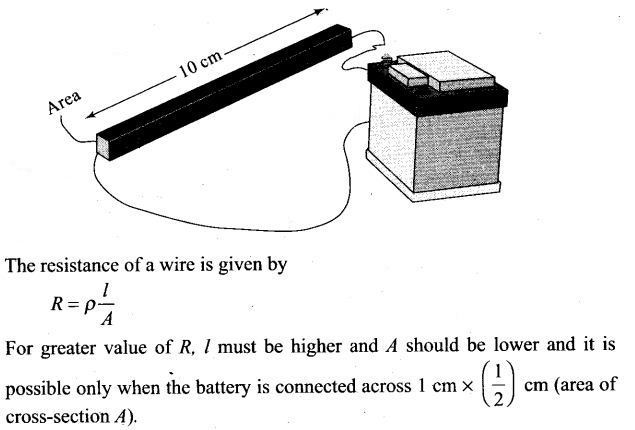Question 6. Which of the following characteristics of electrons determines the current in a conductor?
(a) Drift velocity alone
(b) Thermal velocity alone
(c) Both drift velocity and thermal velocity
(d) Neither drift nor thermal velocity
Solution: (a)
Key concept: Drift velocity is the average uniform velocity acquired by free electrons inside a metal by the application of an electric field which is responsible for the current through it.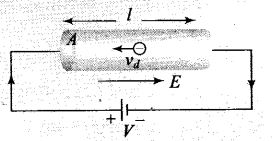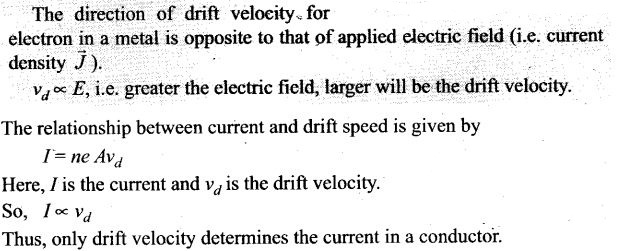Important point: Remember direction of drift velocity and current is opposite, so we are taking the magnitude of drift velocity or drift speed of free electrons.

One or More Than One Correct Answer Type
Question 7. Kirchhoff’s junction rule is a reflection of
(a) conservation of current density vector.
(b) conservation of charge.
(c) the fact that the momentum with which a charged particle approaches a junction is unchanged (as a vector) as the charged particle leaves the junction.
(d) the fact that there is no accumulation of charges at a junction.
Solution: (b, d)
Key concept: Junction rule: At any junction, the sum of the currents entering the junction is equal to the sum of currents leaving the junction.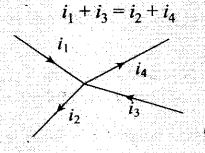Or
Algebraic sum of the currents flowing towards any point in an electric network is zero, i.e., charges are conserved in an electric network.
The proof of this rule follows from the fact that when currents are steady, there is no accumulation of charges at any junction or at any point in a line. Thus, the total current flowing in, (which is the rate at which charge flows into the junction), must equal the total current flowing out.
Kirchhoffs junction rule is also known as Kirchhoff’s current law.
So, Kirchhoffs junction rule is the reflection of conservation of charge
Important point: Sign convention of current from a junction: We are taking outgoing current from a junction as negative. And we are taking incoming current towards a junction as positive.

Question 8. Consider a simple circuit shown in figure stands for a variable resistance R’. R’ can vary from R0 to infinity, r is internal resistance of the battery (r <<R<<R’).
(a) Potential drop across AB is nearly constant as R’ is varied.
(b) Current through R’ is nearly a constant as R’ is varied.
(c) Current I depends sensitively on R’
(d) I ≥ V/r + R always;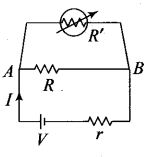Solution: (a, d)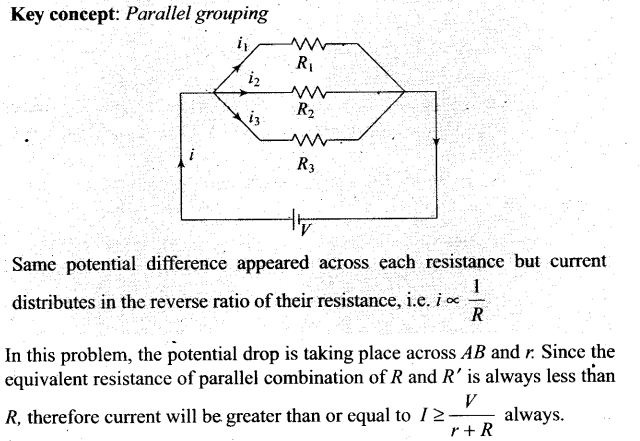Important point: In parallel combination of resistances, the equivalent resistance is smaller than smallest resistance present in combination.

Question 9. Temperature dependence of resistivity ρ(T) of semiconductors, insulators and metals is significantly based on the following factors:
(a) number of charge carriers can change with temperature T.
(b) time interval between two successive collisions can depend on T.
(c) length of material can be a function of T.
(d) mass of carriers is a function of T.
Solution: (a, b) Resistivity is the intrinsic property of the substance.
For a metallic conductor, resistivity is given by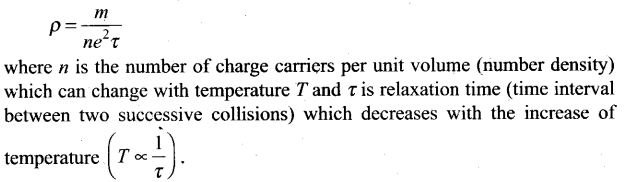Question 10. The measurement of an unknown resistance R is to be carried out using Wheatstone bridge as given in the figure. Two students perform an experiment in two ways. The first student takes R2 = 10 Ω and R1 = 5 Ω. The other student takes R2 = 1000 Ω, and R1 = 500 Ω. In the standard arm, both take R3 = 5 Ω.
Both find R = R2/R1, R3 = 100 Ω within errors.
(a) The errors of measurement of the two students are the same
(b) Errors of measurement do depend on the accuracy with which R2 and R1 can be measured
(c) If the student uses large values of R2 and R1, the currents through the arms will be feeble. This will make determination of null point accurately more difficult.
(d) Wheatstone bridge is a very accurate instrument and has no errors of measurement
Solution: (b, c)
Key concept: Wheatstone bridge:
Wheatstone bridge is an arrangement of four resistance which can be used to measure one of them in terms of rest. Here arms AB and BC are called ratio arm and arms AC and BD are called conjugate arms.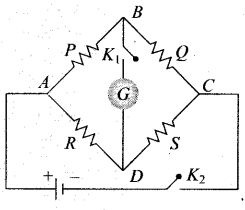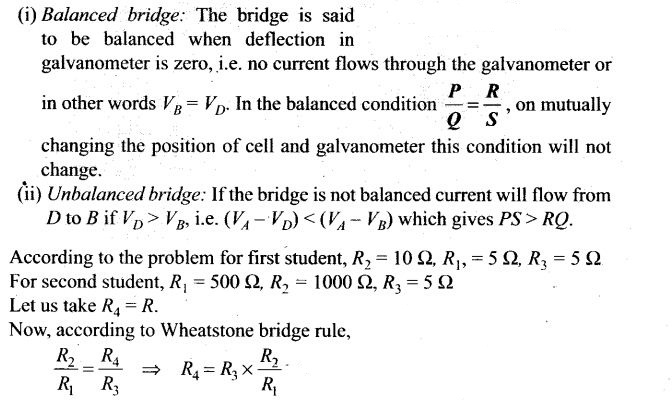Now putting all the values in above equation, we get R = 10 Ω for both students. Thus, we can analyse that the Wheatstone bridge is most sensitive and accurate if resistances are of same value.
Thus, the errors of measurement of the two students depend on the accuracy and sensitivity of the bridge, which in turn depends on the accuracy with which R2 and R1 can be measured.
The currents through the arms of bridge is very weak, when R2 and R1 are larger.
This can make the determination of null point accurately more difficult.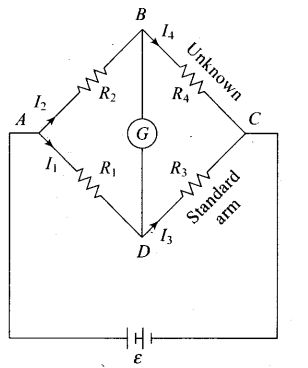Question 11. In a meter bridge, the point D is a neutral point (figure).
(a) The meter bridge can have no other neutral. A point for this set of resistances.
(b) When the jockey contacts a point on meter wire left of D, current flows to B from the wire
(c) When the jockey contacts a point on the meter wire to the right of D, current flows from B to the wire through galvanometer
(d) When R is increased, the neutral point shifts to left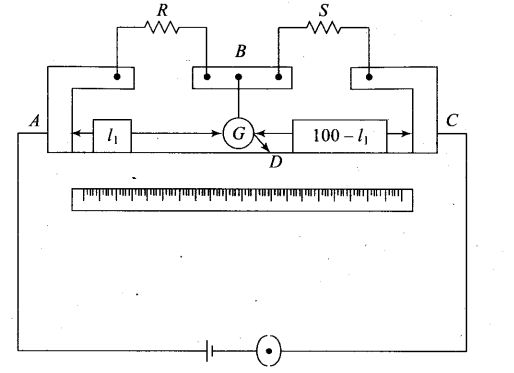Solution: (a, c)
Key concept: Meter bridge: In case of meter bridge, the resistance wire AC is 100 cm long. Varying the position of tapping point B, bridge is balanced. If in balanced position of bridge AB = l, BC = (100 – l) so that Q/P= (100-l)/l. Also P/Q=R/S=>S=(100-l)/l R
When there is no deflection in galvanometer there is no current across the galvanometer, then points B and D are at same potential. That point at which galvanometer shows no deflection is called null point, then potential at B and neutral point D are same. When the jockey contacts a point on the meter wire to the right of D, the potential drop across AD is more than potential drop across AB, which brings the potential of point D less than that of B, hence current flows from B to D in the galvanometer wire.

Very Short Answer Type Questions
Question 12. Is the motion of a charge across junction momentum conserving? Why or why not?
Solution: In the circuit when an electron approaches a junction, in addition to the uniform E that faces it normally (which keep the drift velocity fixed), as drift velocity (vd) is directly proportional to Electric field (E). That’s why there are accumulation of charges on the surface of wires at the junction.
These produce additional electric fields. These fields alter the direction of momentum. Thus, the motion of a charge across junction is not momentum conserving.

Question 13. The relaxation time T is nearly independent of applied field E whereas it changes significantly with temperature T. First fact is (in part) responsible for Ohm’s law whereas the second fact leads to variation of p with temperature. Elaborate why?
Solution: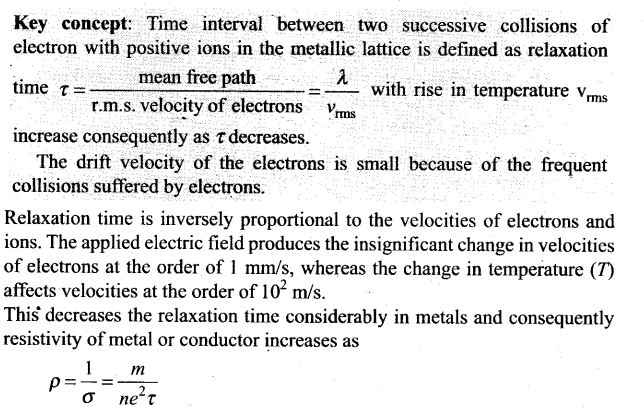Question 14. What are the advantages of the null-point method in a Wheatstone bridge? What additional measurements would be required to calculate R unknown by any other method?
Solution: In a Wheatstone bridge the main advantage of null point method is that the resistance of galvanometer does not affect the balance point, there is no need to determine current in resistances and the internal resistance of a galvanometer. It is convenient and easy method for observer.
The R unknown can calculated applying Kirchhoff’s rules to the circuit. We would need additional accurate measurement of all the currents in resistances and galvanometer and internal resistance of the galvanometer.
Important point: The necessary and sufficient condition for balanced Wheatstone bridge is P/Q = R/S
where P and Q are ratio arms and R is known resistance and S is unknown resistance.

Question 15. What is the advantage of using thick metallic strips to join wires in a potentiometer?
Solution: Metallic strips have negligible resistance and need not to be counted in the length l1, of the null point of potentiometer. That’s why the thick metallic strips are used in potentiometer. It is for the convenience of experimenter as he measures only their lengths along the straight segments each of lengths 1 m.
This measurements is done with the help of a centimetre scale or metre scale and leads to the accurate measurements.

Question 16. For wiring in the home, one uses Cu wires or Al wires. What considerations are involved in this?
Solution: For the selection of metal for wiring in home the main criterion are: the availability, conductivity and the cost of the metal.
The Cu wires or Al wires are used for wiring in the home. The main considerations are involved in this process are cost of metal and good conductivity of metal.

Question 17. Why are alloys used for making standard resistance coils?
Solution: Alloys are used for making standard resistance coil because they have low temperature coefficient of resistance with less temperature sensitivity.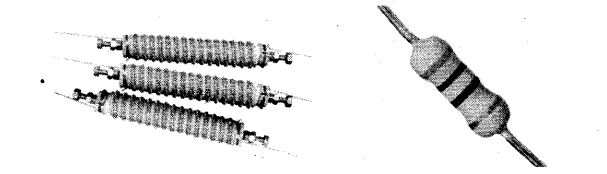This keeps the resistance of the wire almost constant even in small temperature change. The alloys also have high resistivity and hence high resistance, because for given length and cross-section area of conductor (L and A are constant).
R α p

Question 18. Power P is to be delivered to a device via transmission cables having resistance Rc. If V is the voltage across R and I the current through it, find the power wasted and how can it be reduced.
Solution: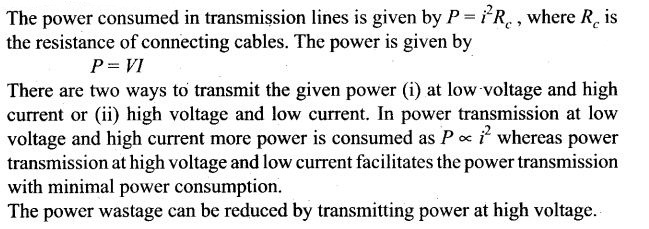Question 19. AB is a potentiometer wire (figure). If the value of R is increased, in which direction will the balance point J shift?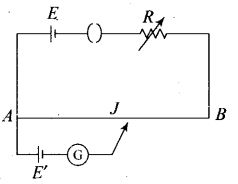Solution: If the value of R is increased, the current through the wire will decrease which in turn decreases the potential difference across AB, and hence potential gradient (k) across AB decreases.
Since, at neutral point, for given emf of cell, l increases as potential gradient (k) across AB has decreased because E’ = kl
Thus, with the increase of l, which will result in increase in balance length. So, jockey J will shift towards B.

Question 20. While doing an experiment with potentiometer (figure) it was found that the deflection is one sided and (i) the deflection decreased while moving from one end A of the wire, to the end R; (ii) the deflection increased, while the jockey was moved towards the end D.
(i) Which terminal positive or negative of the cell E1 is connected at X in case (i) and how is E1 related to E?
(ii) Which terminal of the cell E1 is connected at X in case (ii)?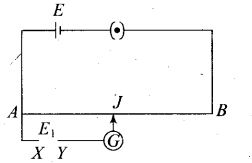Solution: (i) If the current in auxiliary circuit (lower circuit containing primary cell) decreases, and potential difference across A and jockey/increases. Then deflection in galvanometer is one sided and the deflection decreased, while moving from one end ‘A ’ of the wire to the end ‘S’.
And clearly this is possible only when positive terminal of the cell E1 is connected at X and E1>E.
(ii) If the current in auxiliary circuit increases, and potential difference across A and jockey J increases. Then also deflection in galvanometer is one sided.
And this is possible only when negative terminal of the cell E1 is connected at X.

Question 21. A cell of emf E and internal resistance r is connected across an external resistance R. Plot a graph showing the variation of potential difference across R, versus R.
Solution: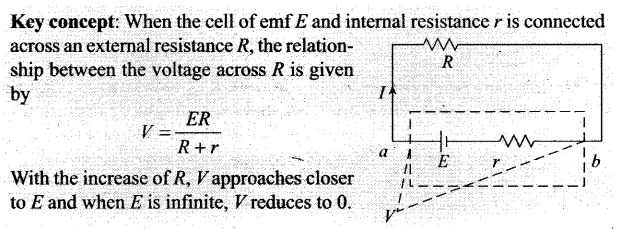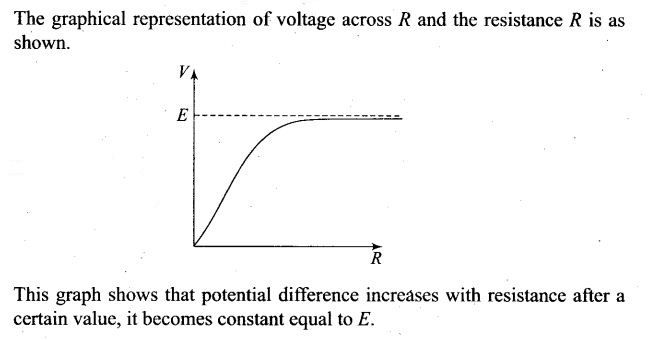Short Answer Type Questions
Question 22. First a set of n equal resistors of R each are connected in series to a battery of emf E and internal resistance R. A current / is observed to flow. Then, the n resistors are connected in parallel to the same battery. It is observed that the current is increased 10 times. What is V?
Solution: Key concept: The equivalent resistance of series combination is in series with the internal resistance R of battery and in parallel combination of resistors, the equivalent resistance of parallel combination is also in series with the internal resistance of battery.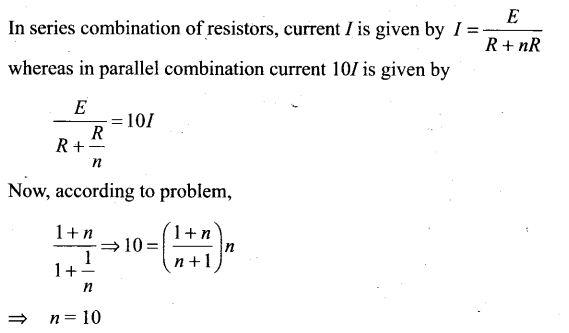Question 23.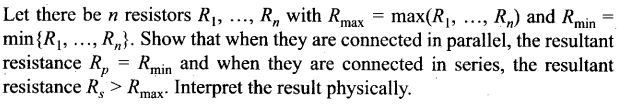Solution: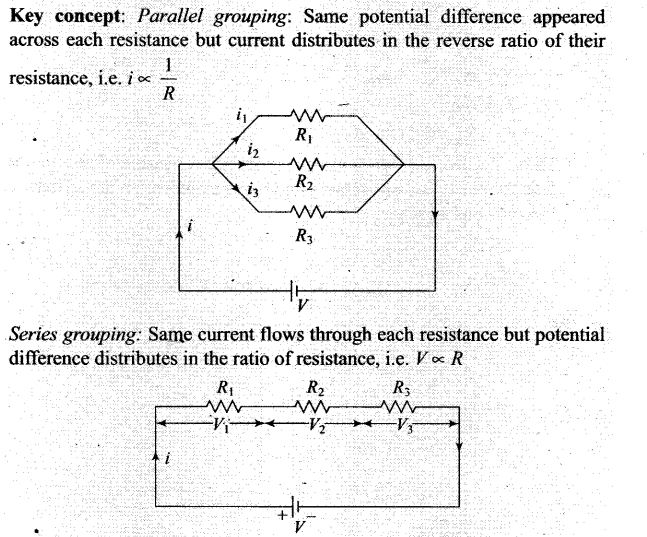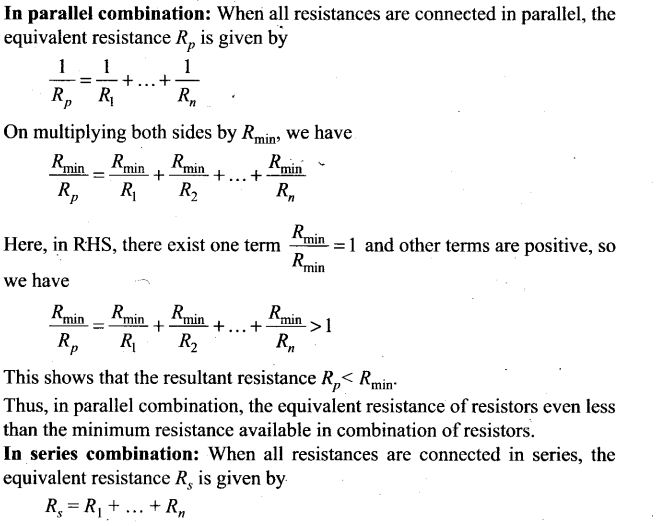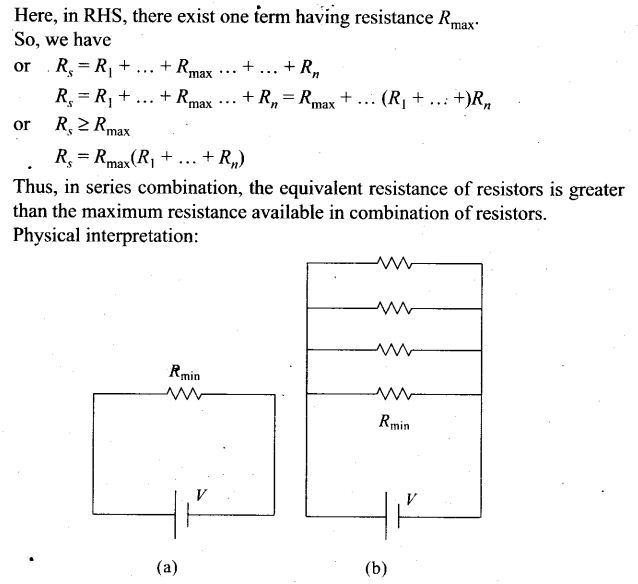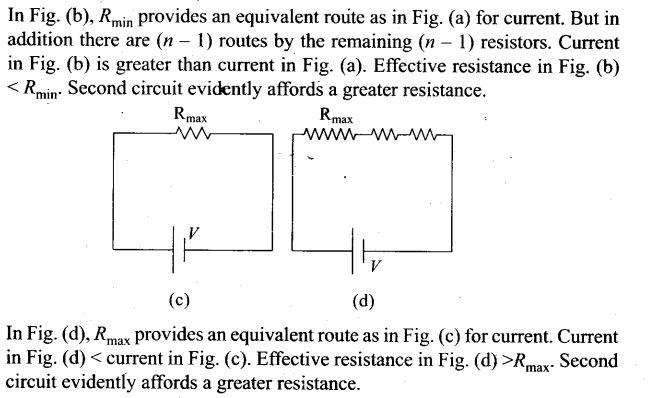Question 24. The circuit in figure shows two cells connected in opposition to each other. Cell E1 is of emf 6 V and internal resistance 2 Ω; the cell E2 is of emf 4 V and internal resistance 8 Ω. Find the potential difference between the points A and B.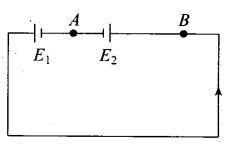Solution: Key concept: In this problem, after finding the electric current flow in the circuit by using Kirchoff’s law or Ohm’s law, the potential difference across AB can be obtained.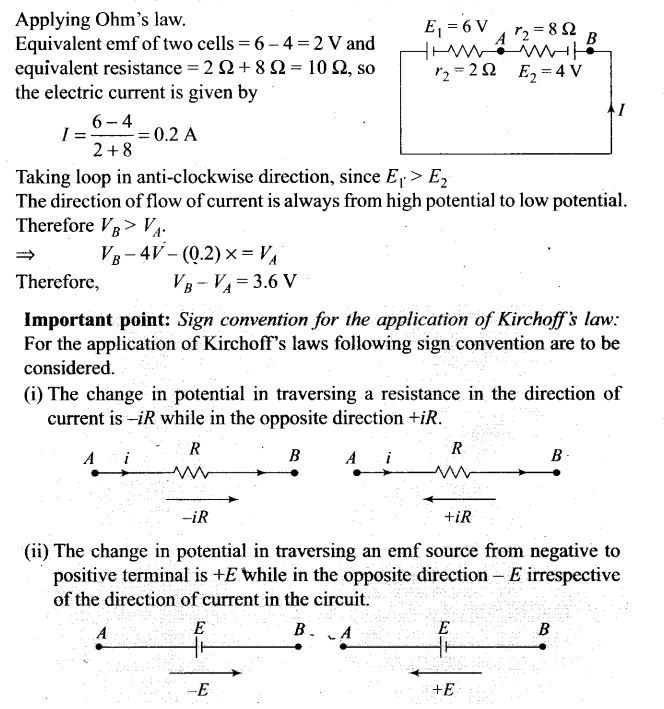Question 25. Two cells of same emf E but internal resistance r1 and r2 are connected in series to an external resistor R (figure). What should be the value of R so that the potential difference across the terminals of the first cell becomes zero?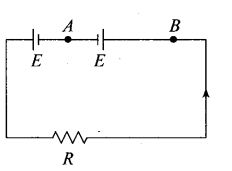Solution: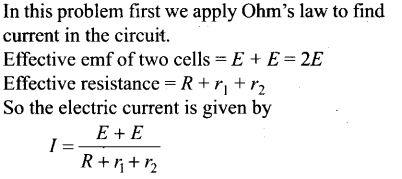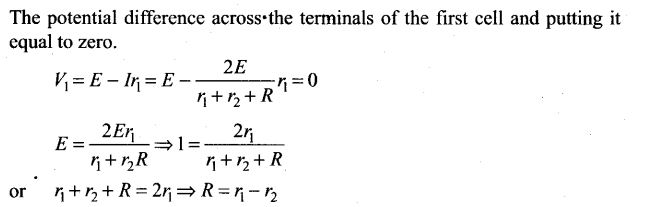Question 26. Two conductors are made of the same material and have the same length. Conductor A is a solid wire of diameter 1 mm. Conductor B is a hollow tube of outer diameter 2 mm and inner diameter 1 mm. Find the ratio of resistance RA to RB.
Solution: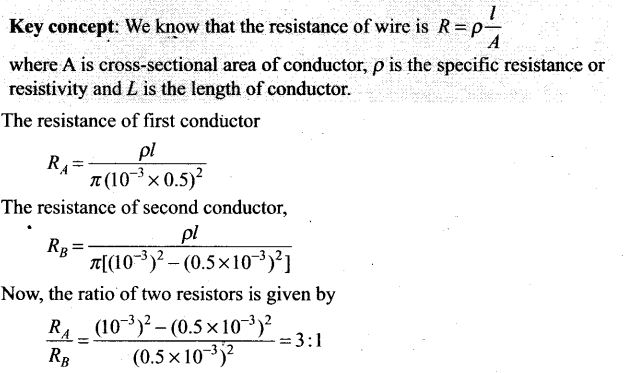Question 27. Suppose there is a circuit consisting of only resistances and batteries. Suppose one is to double (or increase it to n-times) all voltages and all. resistances. Show that currents are unaltered. Do this for circuit of Examples 3, 7 in the NCERT Text Book for Class XII.
Solution: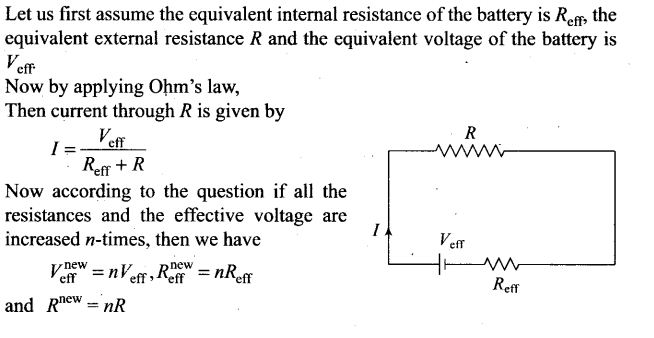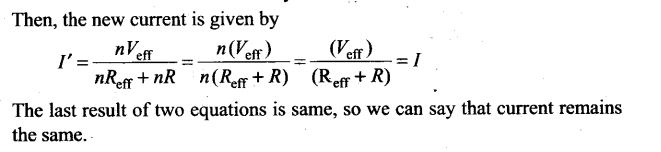Long Answer Type Questions
Question 28. Two cells of voltage 10 V and 2 V, and internal resistances 10 Ω and 5 Ω respectively, are connected in parallel with the positive end of 10 V battery connected to negative pole of 2 V battery (figure). Find the effective voltage and effective resistance of the combination.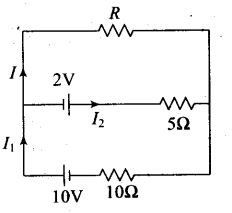Solution: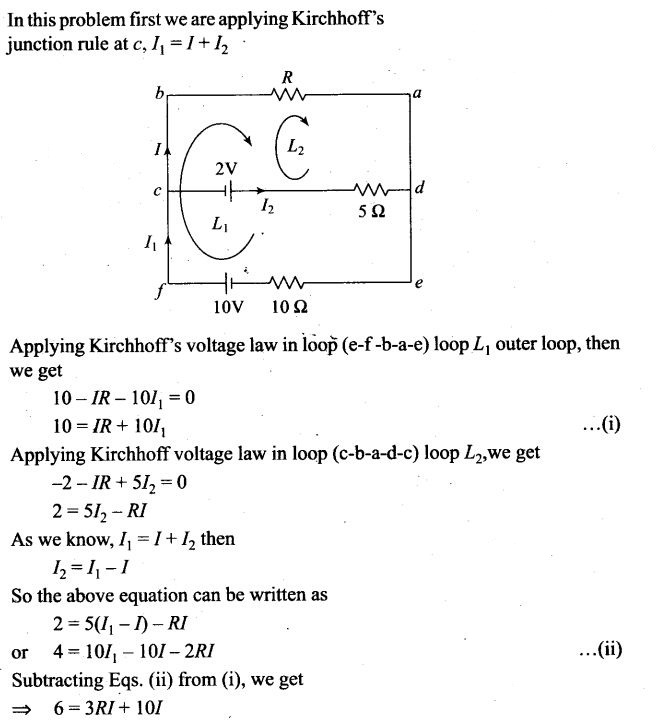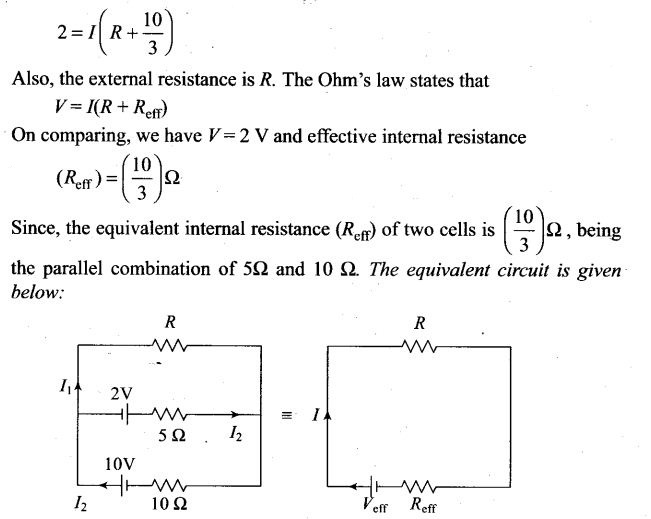Question 29. A room AC runs for 5 a day at a voltage of 220 V. The wiring of the room consists of Cu of 1 mm radius and a length of 10 m. Power consumption per day is 10 commercial units. What fraction of it goes in the joule heating in wires? What would happen if the wiring is made of aluminium of the same dimensions?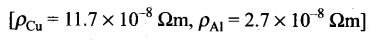Solution: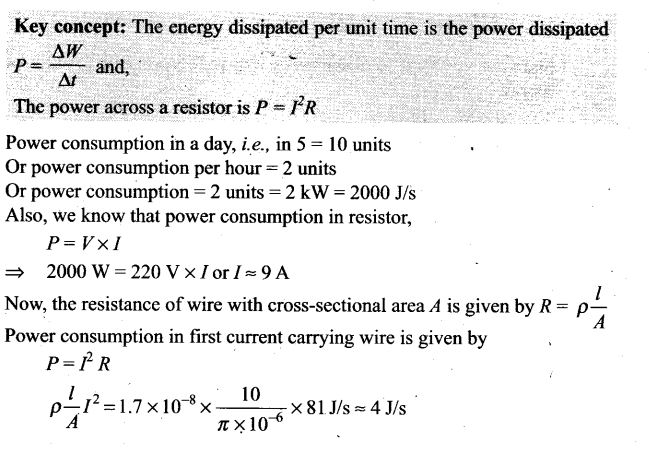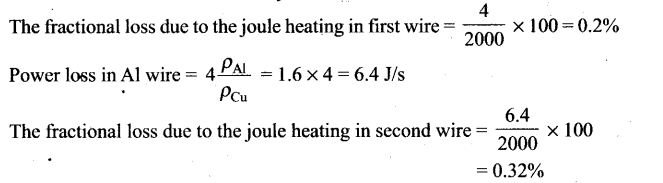Question 30. In an experiment with a potentiometer, VB = 10 V. R is adjusted to be 50 Ω (figure). A student wanting to measure voltage E1 of a battery (approx. 8 V) finds no null point possible. He then diminishes R to 10 Ω and is able to locate the null point on the-last (4th) segment of the potentiometer. Find the resistance of the potentiometer wire and potential drop per unit length across the wire in the second case.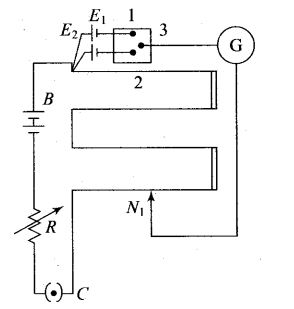Solution: Key concept: When emf of primary cell is less than the potential difference across the wires of potentiometer, only then the null point is obtained.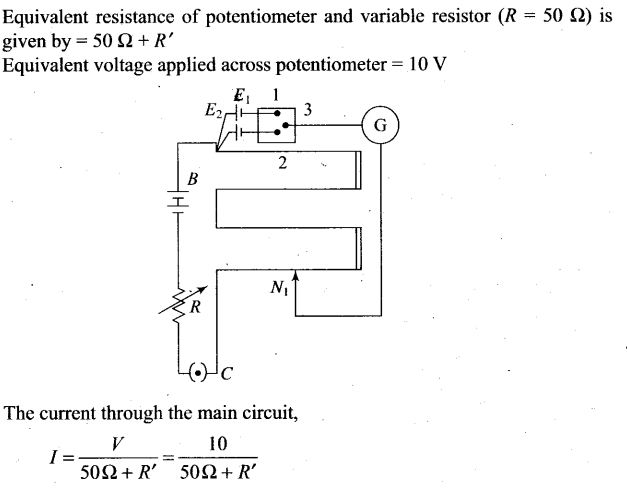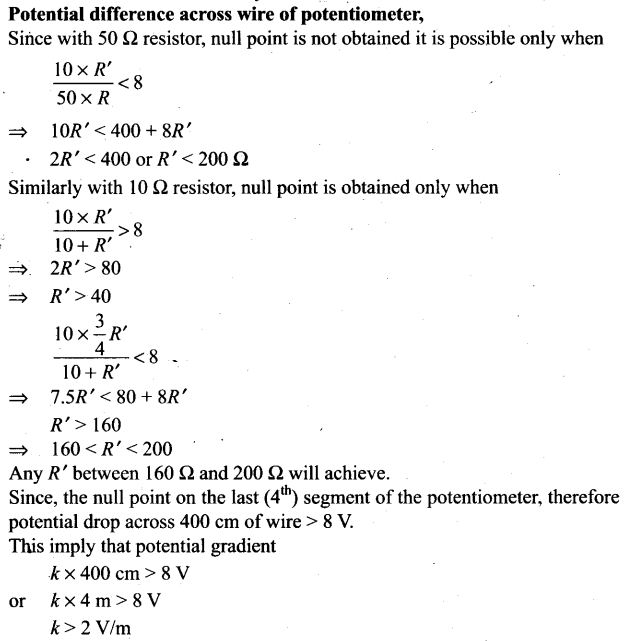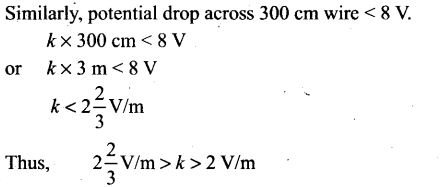Question 31. (a) Consider circuit in figure. How much energy is absorbed by electrons from the initial state of no current (Ignore thermal motion) to the state of drift velocity?
(b) Electrons give up energy at the rate of RI2 per second to the thermal energy. What time scale would number associate with energy in problem (a)? n = number
of electrons/volume = 1029/m3. Length of circuit = 10 cm cross-section . = A = (1 mm)2.
Solution: